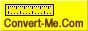## how do you convert a square to a circle

Questions and answers on how to convert things from one unit or system to another
Forum rules
Dear convert-me.com forum visitors,

Our forum has been available for many years. In September 2014 we decided to switch it to read-only mode. Month after month we saw less posts with questions and answers from real people and more spam posts. We were spending more and more resources cleaning the spam until there were less them 1 legitimate message per 100 spam posts. Then we decided it's time to stop.

All the posts in the forum will be available and searchable. We understand there are a lot of useful information and we aren't going to remove anything. As for the new questions, you can always ask them on convert-me.com FaceBook page

Thank you for being with us and sorry for any inconveniences this could caused.

### how do you convert a square to a circle

I am trying to find out what a square turns into a circle. I work in a tubemill factory and I am trying to determine say a 1.5 inch round tube and once it is squeezed into square what size it will be. Is there a formula for this. For the 1.5 square the round tube is 1.9 but I want to know what formula. is used thanks
rpenner

### Re: how do you convert a square to a circle

rpenner wrote:I am trying to find out what a square turns into a circle. I work in a tubemill factory and I am trying to determine say a 1.5 inch round tube and once it is squeezed into square what size it will be. Is there a formula for this. For the 1.5 square the round tube is 1.9 but I want to know what formula. is used thanks

I suppose when squeezing your tube into square the circumference of the initial circle is equal to the perimeter of the square. The formula for the circumference is pi*d, where d is the diameter and pi = 3.14159. The perimeter of a square is 4 times is side.

So pi*d = 4*a, thus d = 4*a/pi = a * 4/3.14159 = a * 1.2732

Checking for a = 1.5, d = 1.5 * 1.2732 = 1.91. So your figures comply to the formula.Again, here it is: d = a * 1.2732. Or a = d * 0.785
Convert-Me.Com - Interactive Units Conversion
http://www.convert-me.com/convert-me.com

Posts: 402
Joined: Fri Sep 05, 2003 4:50 pm